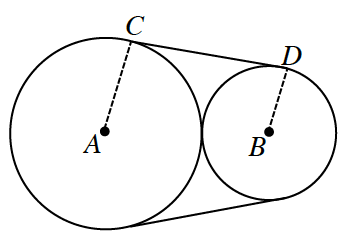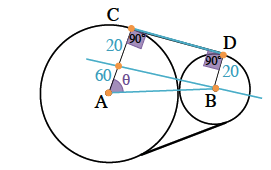### Home > PC > Chapter 6 > Lesson 6.4.1 > Problem6-147

6-147.

Two logs of radius $80$ cm and $20$ cm have a single wire around them as shown in the figure below. Find the length of the wire. Hint: $∠ACD$ and $∠BDC$ are right angles. Draw a line through $B$ parallel to $CD$ meeting $AC$ at $E$.Label the drawing!

Line $AB = 100$ because it is the sum of the radii of both logs.Then $\cosθ = 0.6$, so $θ ≈ 0.927$ radians. Thus the wire length around the large log is $80\left(2π- 2\left(0.927\right)\right) = 354.287$ cm.